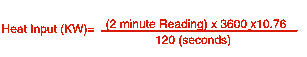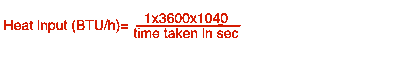Is my boiler using too much gas?

The formula used to gas rate an appliance varies according to the type of meter you have. On metric gas meters write down the reading before and after a 2 minute period of the appliance in question running at max gas consumption, subtract the first from the second, this is the 2 minute reading in the formula.  10.76 is the power in Kw given by 1 cubic meter of gas.On imperial meters you are measuring in cubic feet. One cubic foot of natural gas is one rotation of the test dial and should produce approximately 1040 BTU. Your calculation involves determining how many test dial rotations would occur in an hour. Measure the time for one rotation in seconds and use the following formula.To convert BTU/hour to Kw divide by 3412.

If the figures show 5% above those specified by the manufacturer you have a potentialy dangerous appliance and should call out a Gas Safe registered engineer.

www.heatwizard.co.uk